## Hough Transforms

One powerful global method for detecting edges is called the Hough transform.

Let us suppose that we are looking for straight lines in an image.

If we take a point (x',y') in the image, all lines which pass through that pixel have the form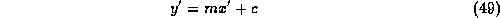for varying values of m and c. See Fig. 30.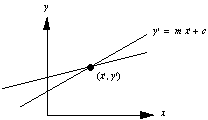Fig. 30 Lines through a point

However, this equation can also be written as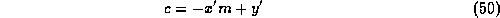where we now consider x' and y' to be constants, and m and c as varying.

This is a straight line on a graph of c against m as shown in Fig. 31.

Each different line through the point (x',y') corresponds to one of the points on the line in (m,c) space.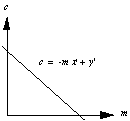Fig. 31 Y = mX + b line model

Now consider two pixels p and q in (x,y) space which lie on the same line.

• For each pixel, all of the possible lines through it are represented by a single line in (m,c) space.
• Thus the single line in (x,y) space which goes through both pixels lies on the intersection of the two lines representing p and q in (m,c) space, as illustrated by Fig. 32.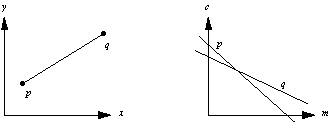Fig. 32 Points on the same line

Taking this one step further,

• All pixels which lie on the same line in (x,y) space are represented by lines which all pass through a single point in (m,c) space.
• The single point through which they all pass gives the values of m and c in the equation of the line y=mx+c.

To detect straight lines in an image, we do:

1. Quantise (m,c) space into a two-dimensional array A for appropriate steps of m and c.
2. Initialise all elements of A(m,c) to zero.
3. For each pixel (x',y') which lies on some edge in the image, we add 1 to all elements of A(m,c) whose indices m and c satisfy y'=mx'+c.
4. Search for elements of A(m,c) which have large values -- Each one found corresponds to a line in the original image.

One useful property of the Hough transform is that the pixels which lie on the line need not all be contiguous.

For example, all of the pixels lying on the two dotted lines in Fig. 33 will be recognised as lying on the same straight line. This can be very useful when trying to detect lines with short breaks in them due to noise, or when objects are partially occluded as shown.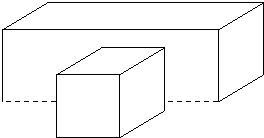Fig. 33 Non-contiguous pixels on the same line

On the other hand, it can also give misleading results when objects happen to be aligned by chance, as shown by the two dotted lines in Fig. 34.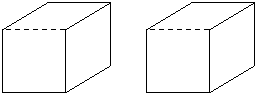Fig. 34 Aligned objects

Indeed, this clearly shows that one disadvantage of the Hough transform method is that it gives an infinite line as expressed by the pair of m and c values, rather than a finite line segment with two well-defined endpoints.

One practical detail is that the y=mx+c form for representing a straight line breaks down for vertical lines, when m becomes infinite.

To avoid this problem, it is better to use the alternative formulation given earlier,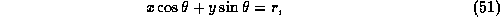as a means of describing straight lines.

Note, however, that a point in (x,y) space is now represented by a curve in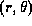space rather than a straight line.

Otherwise, the method is unchanged.

The Hough transform can be used to detect other shapes in an image as well as straight lines.

For example, if we wish to find circles, with equation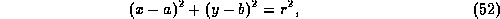Now:

• Every point in (x,y) space corresponds to a surface in (a,b,r) space (as we can vary any two of a, b and r, but the third is determined by Eqn. 53).
• The basic method is, thus, modified to use a three-dimensional array A(a,b,r),
• All points in it which satisfy the equation for a circle are incremented.

In practice, the technique takes rapidly increasing amounts of time for more complicated curves as the number of variables (and hence the number of dimensions of A) increases

So the method is really only of use for simple curves.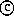David Marshall 1994-1997